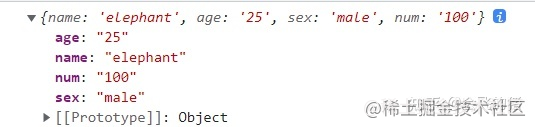﻿ 原生JS获取URL链接参数的几种常见方法_javascript技巧_脚本之家
javascript技巧# 原生JS获取URL链接参数的几种常见方法

## 1. 获取方式总结

• 通过正则匹配的方式
• 利用a标签内置方法
• 利用split方法分割法
• 使用URLSearchParams方法

## 2. 具体实现方法

### 2.1 正则匹配法

```<script>
// 利用正则表达式
let url = "http://www.baidu.com?name=elephant&age=25&sex=male&num=100"
// // 返回参数对象
function queryURLParams(url) {
let pattern = /(\w+)=(\w+)/ig; //定义正则表达式
let parames = {}; // 定义参数对象
url.replace(pattern, (\$, \$1, \$2) => {
parames[\$1] = \$2;
});
return parames;
}
console.log(queryURLParams(url))
</script>```### 2.2 利用a标签```<script>
let URL = "http://www.baidu.com?name=elephant&age=25&sex=male&num=100#smallpig"
function queryURLParams(url) {
// 1.创建a标签
let link = document.createElement('a');
let searchUrl = link.search.substr(1); // 获取问号后面字符串
let hashUrl = link.hash.substr(1); // 获取#后面的值
let obj = {}; // 声明参数对象
// 2.向对象中进行存储
hashUrl ? obj['HASH'] = hashUrl : null; // #后面是否有值

let list = searchUrl.split("&");
for (let i = 0; i < list.length; i++) {
let arr = list[i].split("=");
obj[arr] = arr;
}
return obj;
}
console.log(queryURLParams(URL))
</script>```### 2.3 split分割法

```<script>
let URL = "http://www.baidu.com?name=elephant&age=25&sex=male&num=100"
function queryURLParams(URL) {
// const url = location.search; // 项目中可直接通过search方法获取url中"?"符后的字串
let url = URL.split("?");
let obj = {}; // 声明参数对象
let arr = url.split("&"); // 以&符号分割为数组
for (let i = 0; i < arr.length; i++) {
let arrNew = arr[i].split("="); // 以"="分割为数组
obj[arrNew] = arrNew;
}
return obj;
}
console.log(queryURLParams(URL))
</script>```### 2.4 URLSearchParams方法

URLSearchParams方法能够让我们非常方便的获取URL参数，但是存在一定的兼容性问题，官网的解释如下：

URLSearchParams 接口定义了一些实用的方法来处理 URL 的查询字符串。

```<script>
let URL = "http://www.baidu.com?name=elephant&age=25&sex=male&num=100"
function queryURLParams(URL) {
let url = URL.split("?");
const urlSearchParams = new URLSearchParams(url);
const params = Object.fromEntries(urlSearchParams.entries());
return params
}
console.log(queryURLParams(URL))
</script>```## 附：获取URL携带参数实例

```getUrlParamValue = function (name) {
if (name == null || name == 'undefined') {return null; }
var searchStr = decodeURI(location.search);
var infoIndex = searchStr.indexOf(name + "=");
if (infoIndex == -1) { return null; }
var searchInfo = searchStr.substring(infoIndex + name.length + 1);
var tagIndex = searchInfo.indexOf("&");
if (tagIndex！= -1) { searchInfo = searchInfo.substring(0, tagIndex); }
return searchInfo;
};```